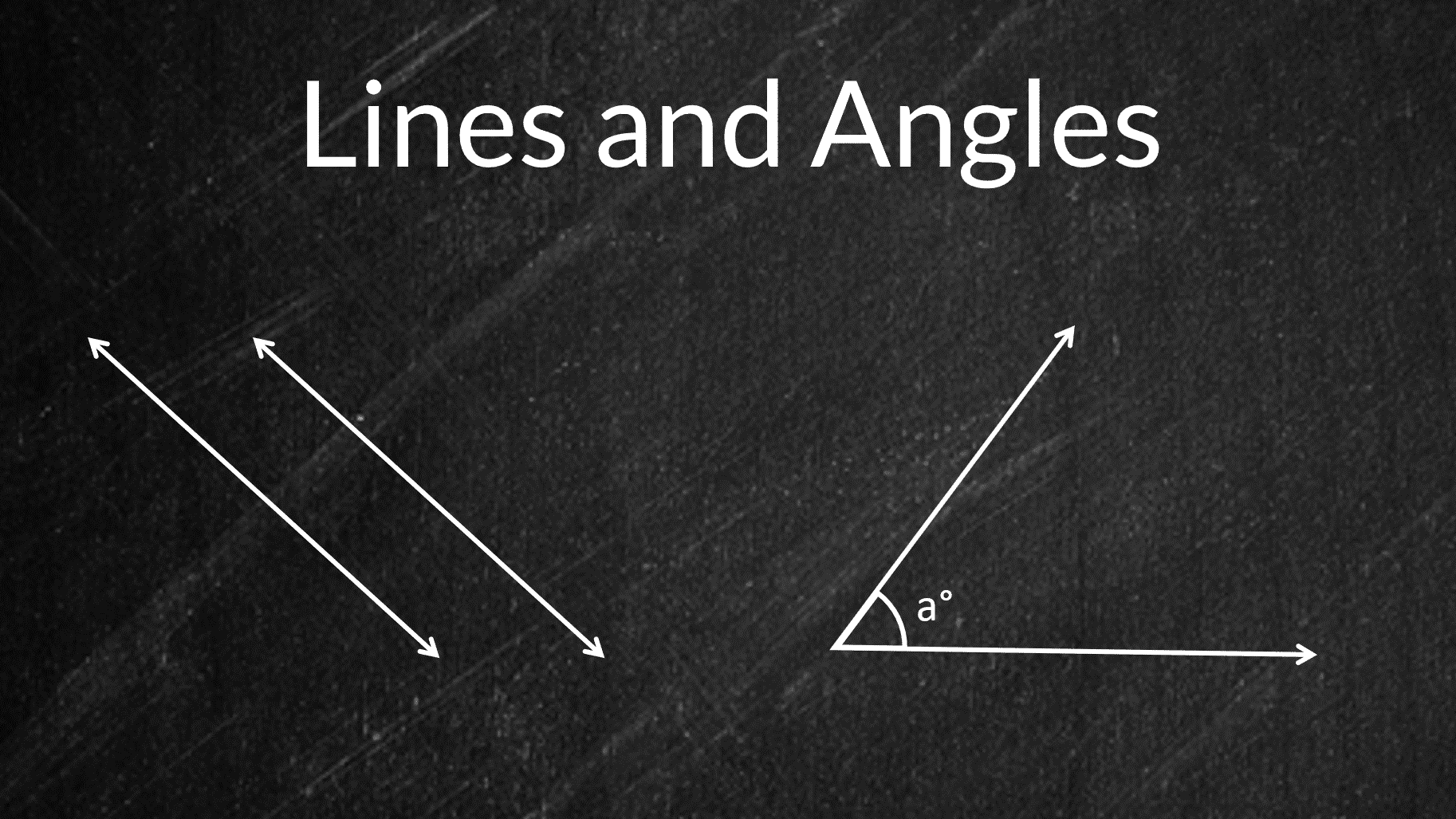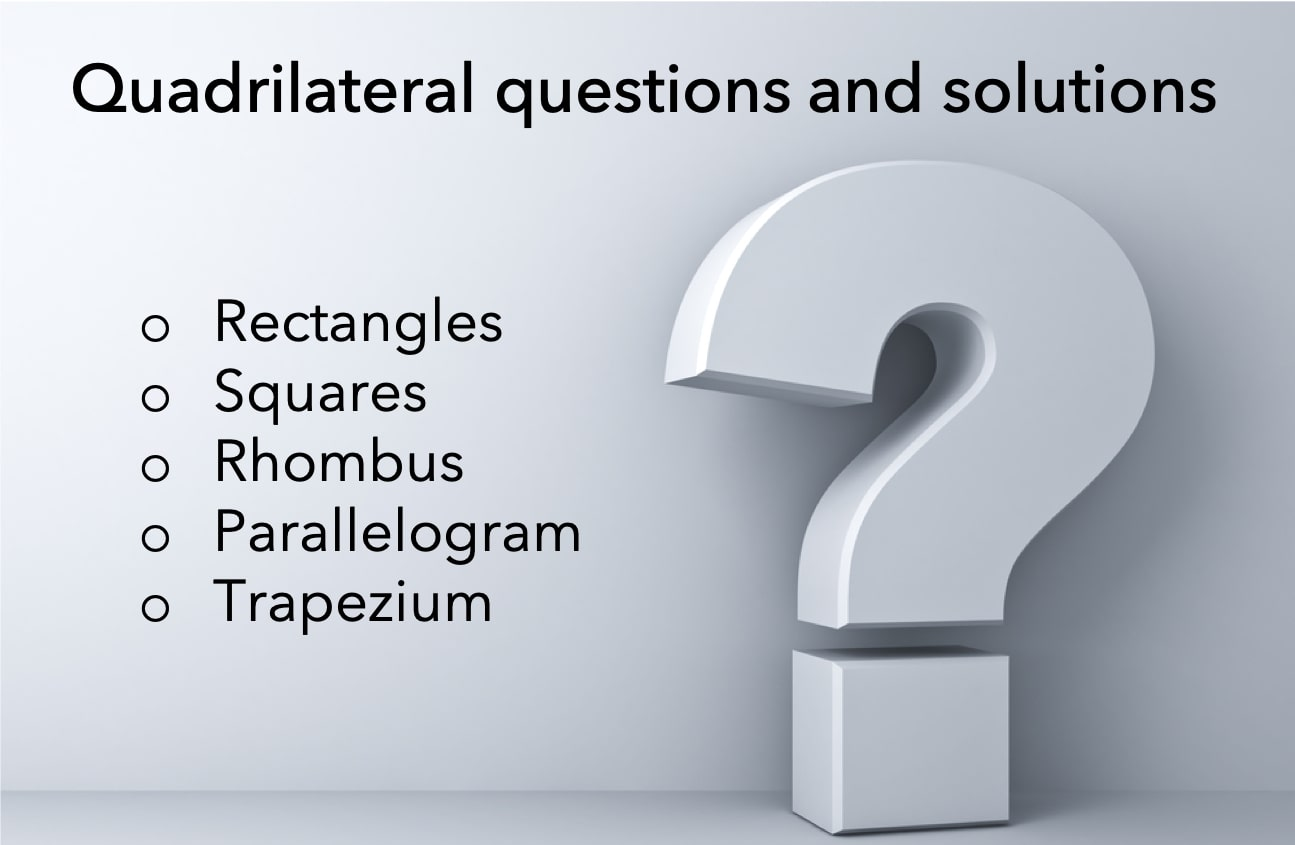# Properties of Quadrilaterals – Rectangle, Square, Parallelogram, Rhombus, Trapezium

In Euclidean geometry, a quadrilateral is a four-sided 2D figure whose sum of internal angles is 360°. The word quadrilateral is derived from two Latin words ‘quadri’ and ‘latus’ meaning four and side respectively. Therefore, identifying the properties of quadrilaterals is important when trying to distinguish them from other polygons. So, what are the properties

Read More# Properties of triangle – Important formulas and classification

In this article, we are going to learn about the simplest form of a polygon, a triangle. All polygons can be divided into triangles, or in other words, they are formed by combining two or more triangles. Thus, understanding the basic properties of triangle and their types is essential. Here is an outline of the

Read More# Lines and Angles – Definitions & Properties (Geometry Tutorial)

Ancient mathematicians introduced the concept of lines to represent straight objects which had negligible width and depth. Considered as a breadth less length by Euclid, lines form the basis of Euclidean geometry. When two rays(part of a straight line) intersect each other in the same plane, they form an angle. The point of intersection is

Read MoreQuadrilateral questions have always been a head-scratcher for a lot of candidates taking competitive or standardized exams. The primary reason behind it is learning a concept is easier as compared to applying that concept to solve questions. Therefore, to help you with the application of properties of quadrilaterals we have shared a few quadrilateral questions

Read More# Properties of Circle | Circle Formulas – Area and Perimeter

A circle is a closed shape formed by tracing a point that moves in a plane such that its distance from a given point is constant. The word circle is derived from the Greek word kirkos, meaning hoop or ring. in this article, we cover the important terms related to circles, their properties, and various

Read More# On a scale drawing, a rectangle 1 inch by 1(1/3) inches represents the floor of a room and the indicated scale is 1-inch equals 15 feet. How many square tiles 6 inches on a side will be needed to cover this floor? (1 foot = 12 inches) – OG 2020 Question #100 with Solution

Solution Given: Dimensions of the rectangular floor = 1 inch by 1(1/3) inches 1 inch = 15 feet 1 feet = 12 inches   To find: The number of square tiles 6 inches on a side will be needed to cover this floor.   Approach and Working: Dimensions of the rectangle in feet= 1 * 15

Read More# In the floor plan of an executive’s beach house above, the north and south walls of the living room are parallel. What is the floor area, in square feet, of the bedroom? – OG 2020 Question #99 with Solution

Solution Given Let us name the point in the diagram as shown below: AE = 30 ft BE = 30 ft CF = 15 ft AD = 30 ft CD = 30 ft   To Find: Area of Bedroom or ∆ACD   Approach and Working: Since EF is parallel to BC, So, two corresponding angles

Read More# Three-fourths of the area of a rectangular lawn 30 feet wide by 40 feet long is to be enclosed by a rectangular fence. If the enclosure has full width and reduced length rather than full length and reduced width, how much less fence will be needed? – OG 2020 Question #98 with Solution

Solution Given In this question, we are given A rectangular lawn is 30 feet wide and 40 feet long. Three-fourth of the area of the lawn is to be enclosed by a rectangular fence.   To Find We need to determine The less amount of fence required, if the enclosure has full width and reduced

Read More# In quadrilateral ABCD above, what is the length of AB? – OG 2020 Question #97 with Solution

Read MoreRead More